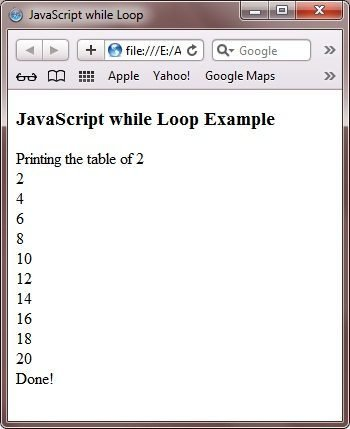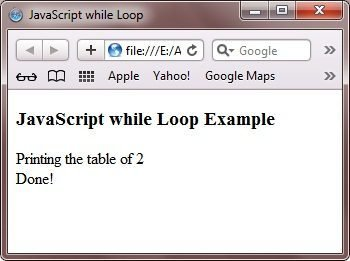# JavaScript while Loop

The while loop in JavaScript is used when you want to check the condition at the start of the loop and then the group of statements that is to be executed is specified after the condition.

Here is the general form to use while loop in JavaScript:

```while(condition-checker)
{
// block of code to be executed here
incrementation;
}```

## JavaScript while Loop Example

Here is an example of while loop in JavaScript:

```<!DOCTYPE HTML>
<html>
<title>JavaScript while Loop</title>
<body>

<h3>JavaScript while Loop Example</h3>
<script type="text/javascript">

var num = 2;
document.write("Printing the table of " + num + "<br/>");
var i = 1;
while(i<=10)
{
document.write(num*i + "<br/>");
i++;
}
document.write("Done!");

</script>

</body>
</html>```

Here is the sample output of the above JavaScript while loop example:Here is another example of while loop in JavaScript:

```<!DOCTYPE HTML>
<html>
<title>JavaScript while Loop</title>
<body>

<h3>JavaScript while Loop Example</h3>
<script type="text/javascript">

var num = 2;
document.write("Printing the table of " + num + "<br/>");
var i = 1;
while(i>10)
{
document.write(num*i + "<br/>");
i++;
}
document.write("Done!");

</script>

</body>
</html>```

Here is the sample output of the above while loop example:Tools
Calculator